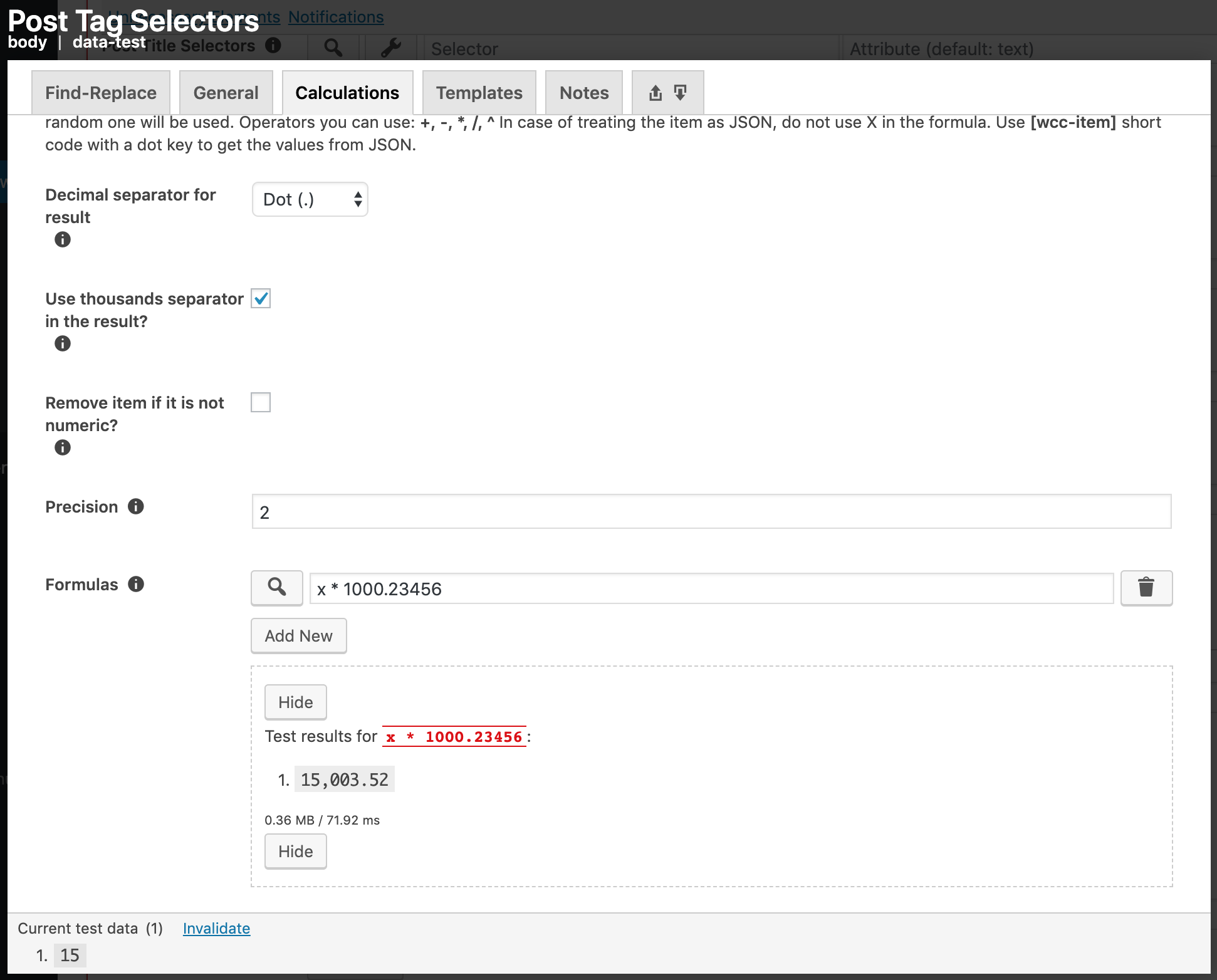# 12.5.2.3. Calculations Tab¶

You can add calculation options for the current value. If there are more than one value, the options will be applied for each value separately. If the item is a number, the formula you enter will be applied to the number. Write X or x to use the current value.

For example, if the current value is 50 and you want to multiply it by 2, then you can write X * 2. You can use parenthesis to group the expressions. For example, ((5 * 7 + 1) / 4^2 - 2) * 10 / X will result in 0.05. Please make sure your mathematical expressions work by using the test button next to each option.

Operators you can use are listed below.

+
Sum two numbers. For example: X + 2 adds 2 to the current value.
-
Subtract a number from another. For example: X - 2 subtracts 2 from the current value.
*
Multiply two numbers. For example: X * 2 multiplies the current value by 2.
/
Divide one number to another. For example: X / 2 divides X to 2.
^
Get power of a number. For example: X^2 gets the square of the current value.

Important

In case of treating the item as JSON (See: Treat as JSON?), do not use X in the formula. Use [wcc-item] short code with a dot key to get the values from JSON.

Note

Options created in Find-Replace Tab and General Tab will be applied first. If you enter more than one formula, a random one will be used.

## 12.5.2.3.1. Decimal separator for result¶

Define the decimal separator for the number that will be shown in your site. When you define the decimal separator, the thousands separator will be the other option. For example, if you select . as decimal separator, , will be the thousands separator.

## 12.5.2.3.2. Use thousands separator in the result?¶

Check this if you want to use thousands separator in the result.

Let’s say the result of a formula entered into Formulas setting is 12000.4 and Decimal separator for result is defined as ., which means the thousands separator is ,. Then, when this is checked, the final result will be 12,000.4. When this is not checked, the final result will be 12000.4.

## 12.5.2.3.3. Remove item if it is not numeric?¶

Check this if you want to remove the item when its value is not numeric. Removing an item means that the plugin will consider there is no item at all. For example, if there are 4 values found but 2 of them are not numbers, the plugin will treat the results as there are only 2 numbers, by discarding the non-numeric ones.

## 12.5.2.3.4. Precision¶

How many digits at max there can be after the decimal separator.

Important

The plugin uses number_format function of PHP to round the numbers. This function rounds to significant figures. You can learn how rounding is done from Significant Figures at Wikipedia.

For example, 4.145 with 2 significant figures will be rounded as 4.15. However, when it is rounded to 1 significant figure, it will be 4.1. If you do not know about significant figures, it is recommended you check out the Wikipedia page.

Let’s say the result of a formula entered into Formulas setting is 3.14159265359. When you set this setting’s value as 3, the result will be 3.142. When you set this as 0, the result will be 3.

## 12.5.2.3.5. Formulas¶

Enter the formulas. If you enter more than one, a random one will be used. This is where you actually make the calculation. You can use button to test the final result. When testing, all of the calculation options is taken into account. So, you can actually test how many decimal places there are or the thousands and decimal separators.Fig. 12.18 An example formula and its test results.

An example of a formula and its test result can be seen in Fig. 12.18. You can see that the result has a thousands separator, which is , because Decimal separator for result is selected as . and Use thousands separator in the result? is checked. Also, the result is rounded to 2 decimal places because of the configuration of Precision setting. x is replaced with 15 because the value found by the setting whose options box is currently open is 15, which is shown at the bottom of the options box, in the test data section.